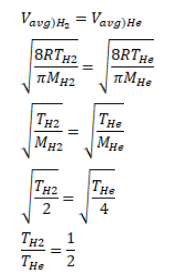# Use R=8.3 J/mol-K wherever required. The mean speed of the molecules of a hydrogen sampleQuestion:

Use $\mathrm{R}=8.3 \mathrm{~J} / \mathrm{mol}-\mathrm{K}$ wherever required.

The mean speed of the molecules of a hydrogen sample equals to the speed of the molecules of helium sample. Calculate the temperature of the hydrogen sample to the temperature of the helium sample.

Solution: